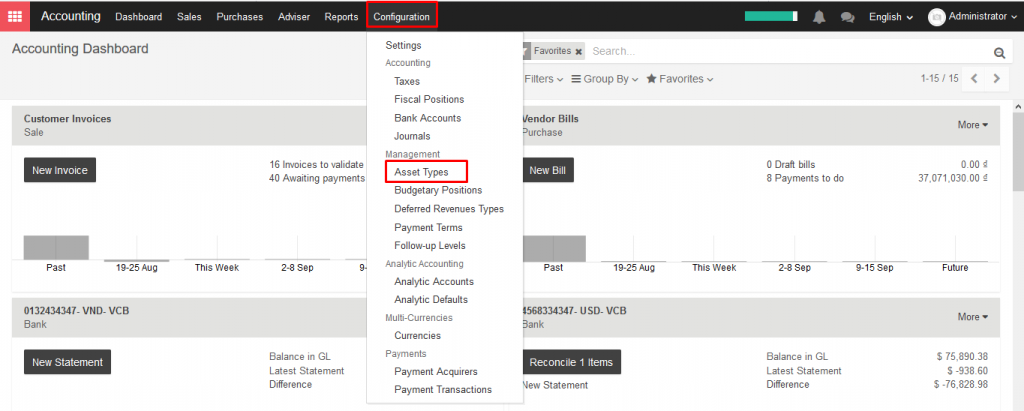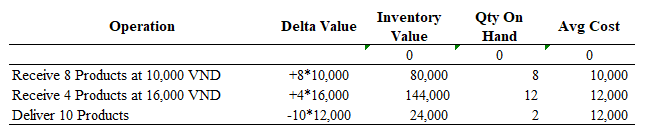# 7.7.1. Impact on the average price valuation when returning goods

As stated in the *inventory valuation page*, one of the possible costing method you can use in perpetual stock valuation, is the average cost.

This document answers to one recurrent question for companies using that method to make their stock valuation: how does a shipping returned to its supplier impact the average cost and the accounting entries? This document is only for the specific use case of a perpetual valuation (as opposed to the periodic one) and in average price costing method (as opposed to standard of FIFO).

→ Definition of average cost

The average cost method calculates the cost of ending inventory and cost of goods sold on the basis of weighted average cost per unit of inventory.

The weighted average cost per unit is calculated using the following formula:

• When new products arrive in a warehouse, the new average cost is re-computed as:

• When products leave the warehouse: the average cost does not change

→ Defining the purchase price

The purchase price is estimated at the reception of the products (you might not have received the vendor bill yet) and reevaluated at the reception of the vendor bill. The purchase price includes the cost you pay for the products, but it may also includes additional costs, like landed costs.

• Average cost example:

At the beginng, the Avg Cost is set to 0 set as there is no product in the inventory. When the first reception is made, the average cost becomes logically the purchase price.

At the second reception, the average cost is updated because the total inventory value is now 80,000 + 4*16,000 = 144,000VND. As we have 12 units on hand, the average price per unit is 144,000 / 12 = 12,000VND.

By definition, the delivery of 10 products does not change the average cost. Indeed, the inventory value is now 24,000 VND as we have only 2 units remaining of each 24,000 / 2 = 12,000 VND.

• Purchase return use case

In case of a product returned to its supplier after reception, the inventory value is reduced using the average cost formulae (not at the initial price of these products!).

Which means that the above table will be updated as follow:

• Explanation: counter example

Remember the definition of Average Cost, saying that we do not update the average cost of a product leaving the inventory. If you break this rule, you may lead to inconsistencies in your inventory.

As an example, here is the scenario when you deliver one piece to the customer and return the other one to your supplier (at the cost you purchased it). Here is the operation:

As you can see in this example, this is not correct: an inventory valuation of 2,000 VND for 0 pieces in the warehouse.

The correct scenario should be to return the goods at the current average cost:

On the other hand, using the average cost to value the return ensure a correct inventory value at all times.

• Further thoughts on anglo saxon mode

For people in using the anglo saxon accounting principles, there is another concept to take into account: the stock input account of the product, which is intended to hold at any time the value of vendor bills to receive. So the stock input account will increase on reception of incoming shipments and will decrease when receiving the related vendor bills.

Back to our example, we see that when the return is valued at the average price, the amount booked in the stock input account is the original purchase price:

This is because the vendor refund will be made using the original purchase price, so to zero out the effect of the return in the stock input in last operation, we need to reuse the original price. The price difference account located on the product category is used to book the difference between the average cost and the original purchase price.